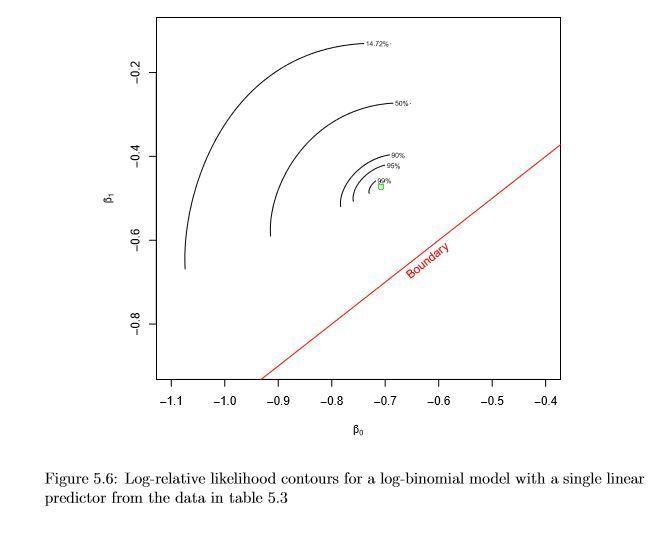## Contour Plot of the Log-Likelihood

Hello everyone, i'm trying to obtain the contour plot of the log-likelihood function of a log-binomial model for some dataset which the log-binomial model fail to obtain the convergence

Here is the code to obtain one of those dataset

data nonconv;
infile datalines dsd;
input Y X;
datalines;
0, -1
0, -1
0, 0
0, 0
0, 1
0, 1
0, 1
0, 1
0, 1
0, 1
0, 1
0, 1
0, 1
0, 1
0, 1
0, 1
0, 1
0, 1
0, 1
1, -1
1, 0
1, 0
1, 0
1, 0
1, 0
1, 0
1, 0
1, 0
1, 0
1, 1
;
And this is the code to obtain the parameter of the model
proc genmod data=nonconv descending;
store logModel;
run;

Unfortunately I can't find how to plot the contour of the log-likelihood, I was trying with the proc plm but i'm not that familiar with this statement.

The plot that i'm trying to obtain is similar to the image below4 REPLIES 4

## Re: Contour Plot of the Log-Likelihood

Are you receiving an error message?

## Re: Contour Plot of the Log-Likelihood

Actually I didn't really understand how the proc pml work, and I don't know if it could help me or if there is any better statement

## Re: Contour Plot of the Log-Likelihood

Your example is for a two-parameter model, so I assume b0=Intercept and b1=parameter for X.

PROC PLM can construct a graph of the predicted values, but SAS regression procedures do not construct plots of the log-likelihood functions. However, you can construct them yourself by writing down the log-likelihood function. Here are some examples that illustrate the method. None of them are exactly what you need, but they show the basic idea about how to do it.:

The basic idea of optimizing a likelihood function

How to create a contour plot in GTL

How to construct a log-likelihood function

Make a contour plot of a probability density function

## Re: Contour Plot of the Log-Likelihood

Remember, PROC SGPLOT supports the VBOX Statement, that will do something similar

Discussion stats
• 4 replies
• 628 views
• 3 likes
• 4 in conversation